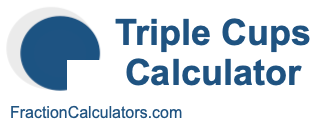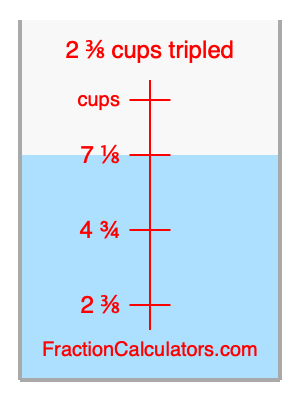What is 2 3/8 cups tripled?Here we will calculate 2 3/8 cups tripled and illustrate 2 3/8 cups tripled on a measuring cup. What is 2 3/8 cups tripled? 2 3/8 cups tripled is 2 3/8 cups times three. Therefore, to triple 2 3/8 cups, we multiply 2 3/8 by 3. Here is the formula, the math, and the answer to 2 3/8 cups tripled:

Cup × 3 = Cup tripled
2 3/8 × 3 = 7 1/8
2 3/8 cups tripled = 7 1/8 cups

Below is an illustration of a measuring cup. We show 2 3/8 cups and 2 3/8 cups tripled (7 1/8 cups) so you can see where they are in relation to each other.As you can see, we filled up our measuring cup to 2 3/8 cups tripled. In other words, we filled it up to 7 1/8, which is three times 2 3/8.

Triple Cups Calculator
Here you can triple another cup measurement.

What is cups tripled?

What is 2 2/5 cups tripled?
Here is the next measurement on our list that we have tripled for you.